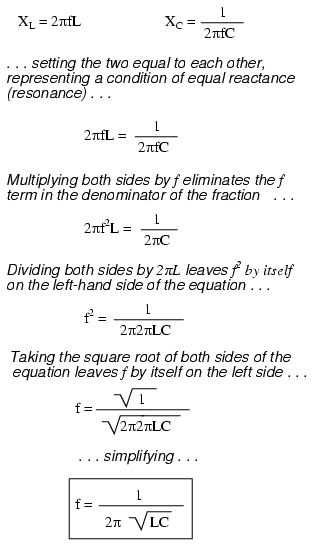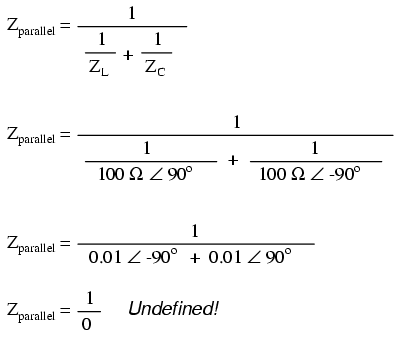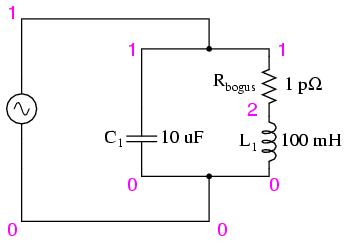6.2: Simple Parallel (Tank Circuit) Resonance

Resonance in a Tank Circuit

A condition of resonance will be experienced in a tank circuit (Figure below) when the reactances of the capacitor and inductor are equal to each other. Because inductive reactance increases with increasing frequency and capacitive reactance decreases with increasing frequency, there will only be one frequency where these two reactances will be equal.Simple parallel resonant circuit (tank circuit).

In the above circuit, we have a 10 µF capacitor and a 100 mH inductor. Since we know the equations for determining the reactance of each at a given frequency, and we’re looking for that point where the two reactances are equal to each other, we can set the two reactance formulae equal to each other and solve for frequency algebraically:So there we have it: a formula to tell us the resonant frequency of a tank circuit, given the values of inductance (L) in Henrys and capacitance (C) in Farads. Plugging in the values of L and C in our example circuit, we arrive at a resonant frequency of 159.155 Hz.

Calculating Individual Impedances

What happens at resonance is quite interesting. With capacitive and inductive reactances equal to each other, the total impedance increases to infinity, meaning that the tank circuit draws no current from the AC power source! We can calculate the individual impedances of the 10 µF capacitor and the 100 mH inductor and work through the parallel impedance formula to demonstrate this mathematically:As you might have guessed, I chose these component values to give resonance impedances that were easy to work with (100 Ω even).

Parallel Impedance Formula

Now, we use the parallel impedance formula to see what happens to total $$Z$$:

$Z_{parallel} = \dfrac{1}{\dfrac{1}{Z_L} + \dfrac{1}{Z_C}}$SPICE Simulation Plot

We can’t divide any number by zero and arrive at a meaningful result, but we can say that the result approaches a value of infinity as the two parallel impedances get closer to each other. What this means in practical terms is that, the total impedance of a tank circuit is infinite (behaving as an open circuit) at resonance. We can plot the consequences of this over a wide power supply frequency range with a short SPICE simulation: (Figure below)Resonant circuit sutitable for SPICE simulation.The 1 pico-ohm (1 pΩ) resistor is placed in this SPICE analysis to overcome a limitation of SPICE: namely, that it cannot analyze a circuit containing a direct inductor-voltage source loop. (Figure below) A very low resistance value was chosen so as to have minimal effect on circuit behavior.

This SPICE simulation plots circuit current over a frequency range of 100 to 200 Hz in twenty even steps (100 and 200 Hz inclusive). Current magnitude on the graph increases from left to right, while frequency increases from top to bottom. The current in this circuit takes a sharp dip around the analysis point of 157.9 Hz, which is the closest analysis point to our predicted resonance frequency of 159.155 Hz. It is at this point that total current from the power source falls to zero.

The “Nutmeg” Graphical Post-Processor Plot

The plot above is produced from the above spice circuit file ( *.cir), the command (.plot) in the last line producing the text plot on any printer or terminal. A better looking plot is produced by the “nutmeg” graphical post-processor, part of the spice package. The above spice ( *.cir) does not require the plot (.plot) command, though it does no harm. The following commands produce the plot below: (Figure below)From the nutmeg prompt:Nutmeg produces plot of current I(v1) for parallel resonant circuit.

Bode Plots

Incidentally, the graph output produced by this SPICE computer analysis is more generally known as a Bode plot. Such graphs plot amplitude or phase shift on one axis and frequency on the other. The steepness of a Bode plot curve characterizes a circuit’s “frequency response,” or how sensitive it is to changes in frequency.

REVIEW:

• Resonance occurs when capacitive and inductive reactances are equal to each other.
• For a tank circuit with no resistance (R), resonant frequency can be calculated with the following formula:• The total impedance of a parallel LC circuit approaches infinity as the power supply frequency approaches resonance.
• A Bode plot is a graph plotting waveform amplitude or phase on one axis and frequency on the other.